# NCERT Class 10 Maths Constructions

The chapter 11 on Constructions deals with the basic constructions and the mathematical reasoning behind them. The first concept that is introduced is to divide a line segment in a given ratio. Then the concept of construction of a triangle similar to a given triangle as per given scale factor is presented. The last section deals with the construction of the tangents to a circle from a point outside it. There is only one exercise in this chapter which has questions based on the constructions discussed in this chapter and the justification for the same.

Download FREE PDF of Chapter-11 Constructions

## Chapter 11 Ex.11.1 Question 1

Draw a line segment of length $$7.6 \,\rm{cm}$$ and divide it in the ratio $$5:8$$. Measure the two parts.

### Solution

#### What is known?

Length of line segment and the ratio to be divided.

Construction

#### Reasoning:

• Draw the line segment of given length.
• Then draw another line which makes an acute angle with the given line.
• Divide the line into $$m + n$$ parts where $$m$$ and $$n$$ are the ratio given.
• Basic proportionality theorem states that, “If a straight line is drawn parallel to a side of a triangle, then it divides the other two sides proportionally".

#### Steps: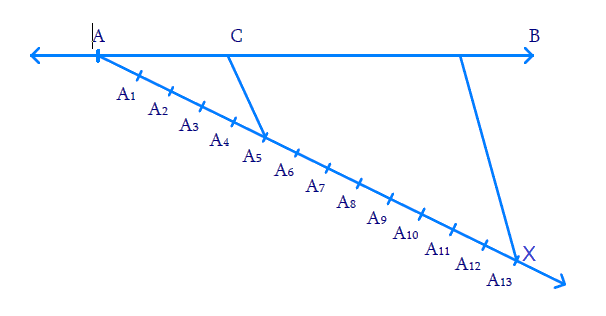(i) Draw $${AB = 7.6\, \rm{cm}}$$

(ii) Draw ray $$AX,$$ making an acute angle width $$AB.$$

(iii) Mark $$13\;(=\;5\;+\;8)$$ points $${A_1},\,{A_2},\,\ldots.\,{A_13}$$ on $$AX$$ such that$$\text{A}{{\text{A}}_{\text{1}}}\text{=}\,{{\text{A}}_{\text{1}}}{{\text{A}}_{\text{2}}}\text{=}{{\text{A}}_{2}}{{\text{A}}_{3}}\text{=}.....{{\text{A}}_{\text{12}}}{{\text{A}}_{\text{13}}}$$

(iv)  Join $${{BA}_{13}}$$

(v) Through $$A_5$$ (since we need $$5$$ parts to $$8$$ parts) draw  $${{C}}{{{A}}_{{5}}}$$ parallel to $${{B}}{{{A}}_{{{13}}}}$$ where $$C$$ lies on $$AB.$$

Now $${{AC: CB = 5:8}}$$

We find $$AC = 2.9 \,\rm{cm}$$ and $$CB = 4.7 \,\rm{cm}$$

Proof:

$${{C}}{{{A}}_{{5}}}$$ is parallel to $${{B}}{{{A}}_{{{13}}}}$$

By Basic Proportionality theorem, in $${{\Delta A}}{{{A}}_{{{13}}}}{{B}}$$

\begin{align}\frac{{AC}}{{CB}} = \frac{{{{A}}{{{A}}_{{5}}}}}{{{{{A}}_{{5}}}{{{A}}_{{{13}}}}}}{{ = }}\frac{{{5}}}{{{8}}}\,\,\end{align} (By Construction)

Thus, $$C$$ divides $$AB$$ in the ratio $$5:8$$.

## Chapter 11 Ex.11.1 Question 2

Construct a triangle of sides $$4 \;\rm{cm}$$, $$5 \;\rm{cm}$$ and $$6\; \rm{cm}$$ and then a triangle similar to it whose sides are \begin{align}\frac{2}{3}\end{align} of the corresponding sides of the first triangle.

### Solution

#### What is known?

Sides of the triangle and the ratio of corresponding sides of $$2$$ triangles.

Construction.

#### Reasoning:

• Draw the line segment of largest length $$6\, \rm{cm}$$. Measure $$5 \;\rm{cm}$$ and $$4 \;\rm{cm}$$ separately and cut arcs from $$2$$ ends of the line segment such that they cross each other at one point. Connect this point from both the ends.
• Then draw another line which makes an acute angle with the given line ($$6\; \rm{cm}$$).
• Divide the line into $$(m + n)$$ parts where $$m$$ and $$n$$ are the ratio given.
• Two triangles are said to be similar if their corresponding angles are equal. They are said to satisfy Angle-Angle-Angle (AAA) Axiom.
• Basic proportionality theorem states that, “If a straight line is drawn parallel to a side of a triangle, then it divides the other two sides proportionally".

#### Steps: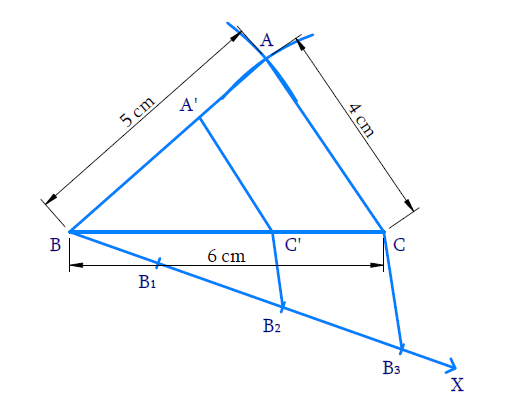Steps of constructions:

(i) Draw $$BC = 6 \text{cm.}$$ With $$B$$ and $$C$$ as centres and radii $$5 \;\rm{cm}$$ and $$4\,\rm{cm}$$ respectively draw arcs to intersect at $$A.$$  $${\rm{\Delta ABC}}$$ is obtained.

(ii) Draw ray $$BX$$ making an acute angle with $$BC.$$

(iii) Mark $$3$$ ( {$$3> 2$$ in the ratio \begin{align}\frac{2}{3}\end{align}points $${{{B}}_{{1}}}{{,}}\,\,{{{B}}_{{2}}}{{,}}\,\,{{{B}}_{{3}}}$$ on $$BX$$ such that $$\,{{B}}{{{B}}_{{1}}}{{ = }}{{{B}}_{{1}}}{{{B}}_{{2}}}{{ = }}{{{B}}_{{2}}}{{{B}}_{{3}}}$$ .

(iv) Join $$\,{{{B}}_{\rm{3}}}{{C}}$$ and draw the line through $${B_2}$$  (2nd point where $$2 < 3$$ in the ratio \begin{align}\frac{2}{3}\end{align}) parallel to $$B_3C$$ meeting $$BC$$ at $${{C'.}}$$

(v) Draw a line thorough $$\,{{C'}}$$ parallel to $$CA$$ to meet $$BA$$ at $$A’.$$ Now $$\,{{\Delta A'BC'}}$$ is the required triangle similar to $$\,{{\Delta ABC}}$$ where

\begin{align}\frac{{{{BC'}}}}{{{{BC}}}}&{{ = }}\frac{{{{BA'}}}}{{{\rm{BA}}}}{\rm{ = }}\frac{{{{C'A'}}}}{{{\rm{CA}}}}\\&{{ = }}\frac{{{2}}}{{{3}}}\end{align}

Proof:

In $$\Delta B B_{3} C, B_{2} C$$ is parallel to $$\,{{{B}}_{{3}}}{{C}} .$$

Hence by Basic proportionality theorem,

\begin{align}\frac{{{{{B}}_{{2}}}{{{B}}_{{2}}}}}{{{{B}}{{{B}}_{{2}}}}}&{{ = }}\frac{{{{C'C}}}}{{{{BC'}}}}\\&{{ = }}\frac{{{1}}}{{{2}}}\end{align}

Adding $$1$$,

\begin{align}\frac{{{{C'C}}}}{{{{BC'}}}}{{ + 1}}& = \frac{{{1}}}{{{2}}}{{ + 1}}\\\frac{{{{C'C + BC'}}}}{{{{BC'}}}}{{ }}&=\frac{{{3}}}{{{2}}}\\\,\,\,\,\,\,\,\,\,\,\,\,\,\,\frac{{{{BC}}}}{{{{BC'}}}}{{}}&= \frac{{{3}}}{{{2}}}\\\,\,\,\,\,\text{(or)}\,\,\,\,\,\,\frac{{{{BC'}}}}{{{{BC}}}}{{ }}&=\frac{{{2}}}{{{3}}} & & {{ \ldots (1)}}\end{align}

Consider $${\rm{\Delta BA'C'}}$$ and $${\rm{\Delta BAC}}$$

$$\angle \text{A }\!\!'\!\!\text{ BC }\!\!'\!\!\text{ }=\angle \text{ABC}$$ (Common)

$$\angle \text{BA }\!\!'\!\!\text{ C }\!\!'\!\!\text{ }=\angle \text{BAC}$$ (Corresponding angles $$∵$$  $$\,\,\text{C }\!\!'\!\!\text{ }A'||\,\text{CA}$$ )

$$\angle \text{BA }\!\!'\!\!\text{ C }\!\!'\!\!\text{ }=\angle \text{BCA}$$ (Corresponding angles $$∵$$ $$\,\,\text{C }\!\!'\!\!\text{ }A'||CA$$ )

Hence by AAA axiom,

${{\Delta BA'C' \sim \Delta BAC}}\,\,$

Corresponding sides are proportional

\begin{align}\frac{{{{BA'}}}}{{{{BA}}}}{{ = }}\frac{{{{C'A'}}}}{{{{CA}}}}{{ = }}\frac{{{{BC'}}}}{{{{BC}}}}{{ = }}\frac{{{2}}}{{{3}}}\,\,\left( {{\text{from}}\,{{(1)}}} \right)\end{align}

## Chapter 11 Ex.11.1 Question 3

Construct a triangle with sides $$5\, \rm{cm},$$ $$6\, \rm{cm}$$ and $$7 \,\rm{cm}$$ and then another triangle whose sides are \begin{align}\frac{7}{5}\end{align} of the corresponding sides of the first triangle.

### Solution

#### What is known?

Sides of the triangle and the ratio of corresponding sides of $$2$$ triangles.

Construction.

#### Reasoning:

• Draw the line segment of largest length $$7 \,\rm{cm}$$. Measure $$5 \,\rm{cm}$$ and $$6 \,\rm{cm}$$ separately and cut arcs from $$2$$ ends of the line segment such that they cross each other at one point. Connect this point from both the ends.
• Then draw another line which makes an acute angle with the given line ($$7 \,\rm{cm}$$).
• Divide the line into $$m + n$$ parts where $$m$$ and $$n$$ are the ratio given.
• Two triangles are said to be similar if their corresponding angles are equal. They are said to satisfy Angle-Angle-Angle (AAA) Axiom.
• Basic proportionality theorem states that, “If a straight line is drawn parallel to a side of a triangle, then it divides the other two sides proportionally".

#### Steps: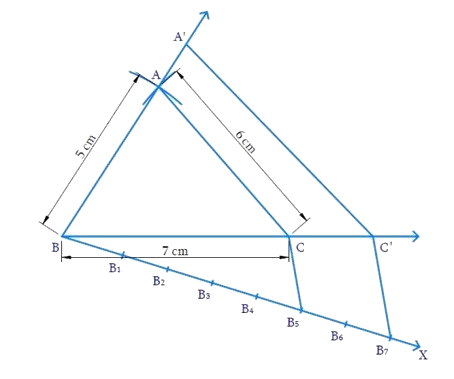Steps of construction:

(i) Draw $${{BC = 7}}\,{\rm{cm}}$$ with $$B$$ and $$C$$ as centres and radii $$5\, \rm{cm}$$ and $$6 \,\rm{cm}$$ respectively. Draw arcs to intersect at $$A.$$ $$\,\Delta {{ABC}}$$ is obtained.

(ii) Draw ray $$BX$$ making $$\,\angle {{CBX}}$$ acute.

(iii) Mark $$7$$ points (greater of $$7$$ and $$5$$ in \begin{align}\frac{7}{5}\end{align} ) $${{{B}}_{{I}}}{{,}}\,{{{B}}_{{2}}}{{,}}\,{{ \ldots \ldots \ldots }}{{{B}}_{{7}}}$$ on $$BX$$ such that $$\,{{B}}{{{B}}_{{I}}}{{ = }}{{{B}}_{{I}}}{{{B}}_{{2}}}{{ = \ldots \ldots \ldots \ldots = }}{{{B}}_{{6}}}{{{B}}_{{7}}}$$

(iv) Join $${{{B}}_{{5}}}$$ (smaller of $$7$$ and $$5$$ in \begin{align}\frac{7}{5}\end{align} and so the $$5^\rm{th}$$ point) to $$C$$ and draw $${{{B}}_{{7}}}{{C'}}$$ parallel to $${{{B}}_{{5}}}{{C}}$$ intersecting the extension of $$BC$$ at  $$C'$$.

(v) Through $${{C'}}$$ draw $${{C'A'}}$$ parallel to $$CA$$ to meet the extension of $$BA$$ at $$A’.$$

Now, $$\,{{\Delta A'}}\,{{B'}}\,{{C'}}$$ is the required triangle similar to $$\,{{\Delta ABC}}$$ where

\begin{align}\frac{{{{BA'}}}}{{{{BA}}}}{{ = }}\frac{{{{C'A'}}}}{{{{CA}}}}{{ = }}\frac{{{{BC'}}}}{{{{BC}}}}{{ = }}\frac{{{7}}}{{{5}}}\end{align}

Proof:

In $$\Delta {B}{B}_{7}{C',}\;{B}_{3}{C}$$ is parallel to $${B}_{7}{C'}$$

Hence by Basic proportionality theorem,

\begin{align}\frac{{{{{B}}_{{6}}}{{{B}}_{{7}}}}}{{{{B}}{{{B}}_{{5}}}}}&{{ = }}\frac{{{{CC'}}}}{{{{BC}}}}\\&{{ = }}\frac{{{2}}}{{{5}}}\end{align}

Adding $$1$$,

\begin{align}\frac{{{{CC'}}}}{{{{BC}}}}{{ + 1}}& = \frac{{{2}}}{{{5}}}{{ + 1}}\\\frac{{{{BC + CC'}}}}{{{{BC}}}}{{ }}&=\frac{{{7}}}{{{5}}}\\\frac{{{{BC'}}}}{{{{BC}}}}{{ }}&= \frac{{{7}}}{{{5}}}\end{align}

Consider $${{\Delta BAC}}$$ and $${{\Delta BA'C'}}$$

$$\angle {{ABC}} = \angle {{A'BC'}}$$ (Common)

$$\angle \text{BCA}\!\!\text{ }=\angle \text{BC}\!'\text{A}\!\!'\!$$ (Corresponding angles $$∵$$ $$\text{CA}||\,\text{C }\!\!'\!\!\text{ }A'\$$ )

$$\angle {{BAC}} = \angle {{BA'C'}}$$ (Corresponding angles)

By AAA axiom, $$\Delta {{BAC}} \sim \Delta {{BA'C'}}$$

$$∴$$  Corresponding sides are proportional

Hence,

\begin{align}\frac{{{{BA'}}}}{{{{BA}}}}{{ = }}\frac{{{{C'A'}}}}{{{{CA}}}}{{ = }}\frac{{{{BC'}}}}{{{{BC}}}}{{ = }}\frac{{{7}}}{{{5}}}\end{align}

## Chapter 11 Ex.11.1 Question 4

Construct an isosceles triangle whose base is $$8 \;\rm{cm}$$ and altitude $$4 \;\rm{cm}$$ and then another triangle whose sides are \begin{align}1\frac{1}{2} \end{align} times the corresponding sides of the isosceles triangle.

### Solution

#### What is known?

Base and altitude of an isosceles triangle and the ratio of corresponding sides of $$2$$ triangles.

Construction.

#### Reasoning:

• Draw the line segment of base $$8 \;\rm{cm}$$. Draw perpendicular bisector of the line. Mark a point on the bisector which measures $$4 \;\rm{cm}$$ from the base. Connect this point from both the ends.
• Then draw another line which makes an acute angle with the given line. Divide the line into $$m + n$$ parts where $$m$$ and $$n$$ are the ratio given.
• Two triangles are said to be similar if their corresponding angles are equal. They are said to satisfy Angle-Angle-Angle (AAA) Axiom.
• Basic proportionality theorem states that, “If a straight line is drawn parallel to a side of a triangle, then it divides the other two sides proportionally".

#### Steps: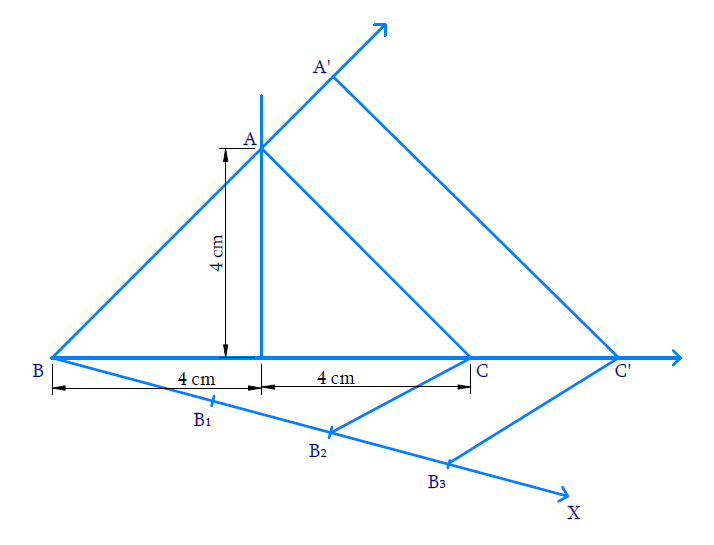Steps of construction:

(i) Draw $$\,{{BC = 8}}\,{\rm{cm}}{{.}}$$ Through $$D,$$ the mid-point of $$BC,$$ draw the perpendicular to $$BC$$ and cut an arc from $$D$$ on it such that $${{DA = 4}}\,{{cm}}{{.}}$$ Join $$BA$$ and $$CA.$$ $${{\Delta ABC}}$$ is obtained.

(ii) Draw the ray $$BX$$ so that $$\angle {{CBX}}$$ is acute.

(iii) Mark $$3$$ \begin{align}\left( {3{{ }} > {{ }}\;2\text{ in}\,\,1\frac{1}{2} = \,\frac{3}{2}} \right)\end{align}  points $${{{B}}_{{I}}}{{,}}\,{{{B}}_{{2}}}{{,}}\,{{{B}}_{{3}}}$$ on BX such that $${{B}}{{{B}}_{{1}}}{{ = }}{{{B}}_{{1}}}{{{B}}_{{2}}}{{ = }}{{{B}}_{{2}}}{{{B}}_{{3}}}$$

(iv) Join $${B}_{2}$$ ($$\;2^\rm{nd}$$  point $$∵ \;2<3$$) to C and draw $${B}_{3} {C}^{\prime}$$ parallel to $${B}_{2} {C}$$ intersect $$BC$$ extended at $$C’$$.

(v)  Through $$C’$$ draw $${{C'A'}}$$ parallel to $$CA$$ to intersect $$BA$$ extended to $$A’.$$

Now, $${{\Delta A'BC'}}$$ is the required triangle similar to $$\,{{\Delta ABC}}$$ where

\begin{align}\frac{{{{BA'}}}}{{{{BA}}}}{{ = }}\frac{{{{C'A'}}}}{{{{CA}}}}{{ = }}\frac{{{{BC'}}}}{{{{BC}}}}{{ = }}\frac{{{3}}}{{{2}}}\end{align}

Proof:

In $${{\Delta B}}{{{B}}_{{3}}}{{C',}}\,\,{{{B}}_{{2}}}{{C}}\,\,{{||}}\,\,{{{B}}_{{3}}}{{C'}}$$ ,

Hence by Basic proportionality theorem,

\begin{align}\frac{{{{{B}}_{{2}}}{{{B}}_{{3}}}}}{{{{B}}{{{B}}_{{2}}}}}{{ = }}\frac{{{{CC'}}}}{{{{BC}}}}{{ = }}\frac{{{1}}}{{{2}}}\end{align}

Adding $$1$$,

\begin{align}\frac{{{{CC'}}}}{{{{BC}}}}{{ + 1}}&= \frac{{{1}}}{{{2}}}{{ + 1}}\\\frac{{{{BC + CC'}}}}{{{{BC}}}}{{ }}&=\frac{{{3}}}{{{2}}}\\\frac{{{{BC'}}}}{{{{BC}}}}{{}}&= \frac{{{3}}}{{{2}}}\end{align}

Consider $$\,{{\Delta BAC }}\,{{and }}\,{{\Delta BA'C'}}$$

$$\angle {{ABC}}\, = \,\angle {{A'BC'}}$$ (Common)

$$\angle {BCA}=\angle {BC' } { A '}$$ (Corresponding angles $$\therefore \mathrm{CA} \| \mathrm{C}^{\prime} \mathrm{A}^{\prime}$$

$$\angle {{BAC}}\, = \,\angle {{BA'C'}}$$ (Corresponding angles)

By AAA axiom, $${{\Delta BAC \sim\Delta BA'C'}}$$

$$∴$$ Corresponding sides are proportional

Hence,

\begin{align}\frac{{{{BA'}}}}{{{{BA}}}}{{ = }}\frac{{{{BC'}}}}{{{{BC}}}}{{ = }}\frac{{{{CA'}}}}{{{{CA}}}}{{ = }}\frac{{{3}}}{{{2}}}\end{align}

## Chapter 11 Ex.11.1 Question 5

Draw a triangle $$ABC$$ with side $${{BC = 6 \,\rm{cm,}\;AB = 5\,\rm{cm}}}$$ and $$\angle {{ABC = 6}}{{{0}}^0}.$$  Then construct a triangle whose sides are \begin{align}\frac{3}{2}\end{align} of the corresponding sides of the triangle $$ABC.$$

### Solution

#### What is known?

$$2$$ sides and the angle between them and the ratio of corresponding sides of $$2$$ triangles.

Construction.

#### Reasoning:

• Draw the triangle with the given conditions.
• Then draw another line which makes an acute angle with the base line. Divide the line into $$m + n$$ parts where $$m$$ and $$n$$ are the ratio given.
• Two triangles are said to be similar if their corresponding angles are equal. They are said to satisfy Angle-Angle-Angle (AAA) Axiom.
• Basic proportionality theorem states that, “If a straight line is drawn parallel to a side of a triangle, then it divides the other two sides proportionally".

#### Steps: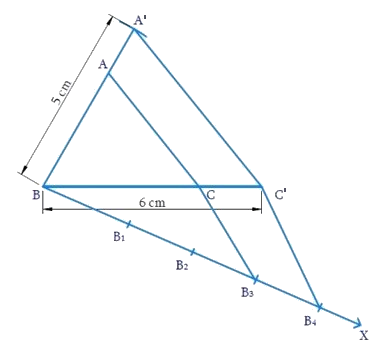Steps of constructions:

(i) Draw $${{BC = 6 \,\rm{cm}}}.$$ At $$B,$$ make $$\angle {{CBY = }}\,{{60^\circ }}$$ and cut an arc at $$A$$ so that $$BA = 5 \,\rm{cm}.$$ Join $$\,{{AC}}\,,\,\,{{\Delta ABC}}$$ is obtained.

(ii) Draw the ray $$BX$$ such that $$\angle {{CBX}}$$ is acute.

(iii) Mark $$4$$ ($${4} > 3$$ in $$\frac{3}{4}$$) points $${{{B}}_1},{{{B}}_{{2}}},\,{{{B}}_{{3}}},\,{{{B}}_{{4}}}$$ on $$BX$$ such that $${{B}}{{{B}}_{{1}}}{{ = }}{{{B}}_{{1}}}{{{B}}_{{2}}}{{ = }}{{{B}}_{{2}}}{{{B}}_{{3}}}{{ = }}{{{B}}_{{3}}}{{{B}}_{{4}}}$$

(iv) Join $${{{B}}_{{4}}}$$ to $$C$$ and draw $${{{B}}_{{3}}}{{C'}}$$ parallel to $${{{B}}_{{4}}}{{C}}$$ to intersect $$BC$$ at $${{C'}}{{.}}$$

(v) Draw $${{C'A'}}$$ parallel to $$CA$$ to intersect $$BA$$ at $$A’.$$

Now, $$\,{{\Delta A'BC'}}$$ is the required triangle similar to $${{\Delta ABC}}$$ where

\begin{align}\frac{{{{BA'}}}}{{{{BA}}}}{{ = }}\frac{{{{BC'}}}}{{{{BC}}}}{{ = }}\frac{{{{C'A'}}}}{{{{CA}}}}{{ = }}\frac{{{3}}}{{{4}}}\end{align}

Proof:

In $${{\Delta }}\,{{B}}{{{B}}_4}{{C'}}\,\,,\,\,{{{B}}_3}{{C' || }}{{{B}}_4}{{C}}$$

Hence by Basic proportionality theorem,

\begin{align}\frac{{{{{B}}_{{3}}}{{{B}}_{{4}}}}}{{{{B}}{{{B}}_{{3}}}}}&= \frac{{{{C'C}}}}{{{{BC'}}}}= \frac{{{1}}}{{{3}}}\\\frac{{{{C'C}}}}{{{{BC'}}}}{{ + 1}}& = \frac{{{1}}}{{{3}}}{{ + 1 \quad \text{(Adding 1)}}}\\\frac{{{{C'C + BC'}}}}{{{{BC'}}}}& = \frac{{{4}}}{{{3}}}\\\frac{{{{BC}}}}{{{{BC'}}}}& =\frac{{{4}}}{{{3}}}\,\, {\rm{(or)}} \,\frac{{{{BC'}}}}{{{{BC}}}} =\frac{{{3}}}{{{4}}}\end{align}

Consider $${{\Delta BA'C'}}\,\,{{and}}\,\,{{\Delta BAC}}$$

\begin{align} & \angle \text{A }\!\!'\!\!\text{ BC }\!\!'\!\!\text{ }=\angle \text{ABC}={{60}^{\circ }} \\ & \angle \text{BCA }\!\!'\!\!\text{ }=\angle \text{BCA}\,\,\\&\left( \text{Corresponding}\,\text{angles}\because \,\text{CA}||\text{CA} \right) \\ & \angle \text{BA }\!\!'\!\!\text{ C }\!\!'\!\!\text{ }=\angle \text{BAC}\\&\left( \text{Corresponding}\,\text{angles} \right) \\ \end{align}

By AAA axiom, $${{\Delta BA'C' \sim \Delta BAC}}$$

Therefore corresponding sides are proportional,

\begin{align}\frac{{{{BC'}}}}{{{{BC}}}}{{ = }}\frac{{{{BA'}}}}{{{{BA}}}}{{ = }}\frac{{{{C'A'}}}}{{{{CA}}}}{{ = }}\frac{{{3}}}{{{4}}}\end{align}

## Chapter 11 Ex.11.1 Question 6

Draw a triangle ABC with side \begin{align}{{BC }} = {{ }}7\,{\rm{ cm,}}\,\,\angle {{B }} = {{ }}45^\circ ,\,\,\angle {{A }} = {{ }}105^\circ .\end{align} Then, construct a triangle whose sides are \begin{align}\frac{4}{3}\end{align} times the corresponding sides of \begin{align}\Delta{ ABC}\end{align}.

### Solution

#### What is known?

One side and $$2$$ angles of a triangle and the ratio of corresponding sides of $$2$$ triangles.

Construction.

#### Reasoning:

• Draw the triangle with the given conditions.
• Then draw another line which makes an acute angle with the base line. Divide the line into $$m + n$$ parts where $$m$$ and $$n$$ are the ratio given.
• Two triangles are said to be similar if their corresponding angles are equal. They are said to satisfy Angle-Angle-Angle (AAA) Axiom.
• Basic proportionality theorem states that, “If a straight line is drawn parallel to a side of a triangle, then it divides the other two sides proportionally".

#### Steps: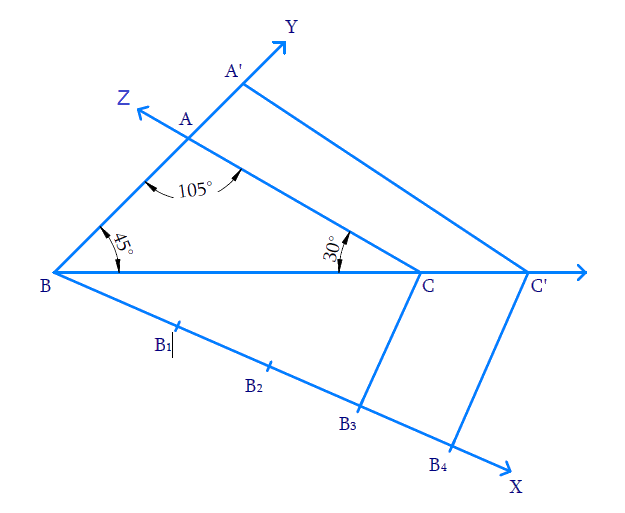Steps of construction:

(i) Draw $${{BC = 7}}\,{\rm{cm}}{{.}}$$ At $$B,$$ make an angle $$\angle {{CBY}} = {45^ \circ }$$ and at $$C,$$ make $$\angle {{BCZ}} = 30^\circ \,\,\left[ {{{180}^ \circ } - \left( {{{45}^ \circ } + {{105}^ \circ }} \right)} \right]$$ . Both $$BY$$ and $$CZ$$ intersect at $$A$$ and thus $${{\Delta ABC}}$$ is constructed.

(ii) Draw the ray $$BX$$ so that $$\angle {{CBX}}$$ is acute.

(iii) Mark $$4$$ \begin{align}\left( {4{{ }} > {{ }}3\,{\rm{ in}}\,\,\frac{4}{3}} \right)\end{align} points $${{{B}}_{{1}}}{{,}}{{{B}}_{{2}}}{{,}}{{{B}}_{{3}}}{{,}}{{{B}}_{{4}}}$$ on BX such that $${{B}}{{{B}}_{{1}}}{{ = }}{{{B}}_{{1}}}{{{B}}_{{2}}}{{ = }}{{{B}}_{{2}}}{{{B}}_{{3}}}{{ = }}{{{B}}_{{3}}}{{{B}}_{{4}}}$$

(iv) Join $$B_3$$ (third point on $$BX,\;3\, < 4$$ in \begin{align}\frac{4}{3}\end{align}) to $$C$$ and draw $${{{B}}_{{4}}}{{C'}}$$ parallel to $$BC$$ such that $$C’$$ lies on the extension of $$BC.$$

(v) Draw $$C’A’$$ parallel to $$CA$$ to intersect the extension of $$BA$$ at $$A’.$$

Now, $$\Delta {{A'BC'}}$$ is the required triangle similar to $${{\Delta ABC}}$$ where,

\begin{align}\frac{{{{BA'}}}}{{{{BA}}}}{{ = }}\frac{{{{BC'}}}}{{{{BC}}}}{{ = }}\frac{{{{C'A'}}}}{{{{CA}}}}{{ = }}\frac{{{4}}}{{{3}}}\end{align}

Proof:

In $${{\Delta B}}{{{B}}_{{4}}}{{C',}}\,\,{{{B}}_{{3}}}{{C}}\,\,{{||}}\,\,{{{B}}_{{4}}}{{C'}}$$

Hence by Basic proportionality theorem,

\begin{align}\frac{{{{{B}}_{{3}}}{{{B}}_{{4}}}}}{{{{B}}{{{B}}_{{3}}}}}&= \frac{{{{CC'}}}}{{{{BC}}}}{{ = }}\frac{{{1}}}{{{3}}}\\\frac{{{{CC'}}}}{{{{BC}}}}{{ + 1 }}&=\frac{{{1}}}{{{3}}}{{ + 1}}\,\quad{\rm{(Adding}}\,{{1)}}\\\frac{{{{BC + CC'}}}}{{{{BC}}}}& = \frac{{{4}}}{{{3}}}\\\frac{{{{BC'}}}}{{{{BC}}}}& = \frac{{{4}}}{{{3}}}\end{align}

Consider $$\Delta BA'C'$$ and  $$\Delta BAC$$

$$\angle {{A'BC'}}\, = \angle {{ABC}} = 45^\circ$$

$$\angle {{BC'A'}} = \angle {{BCA}} = 30^\circ$$ (Correspomding angles as $$\,\,{{CA}}\,{{||}}\,\,{{C'A'}}$$ )

$$\angle {{BA'C'}} = \angle {{BAC}} = 105^\circ$$ (Corresponding angles )

By AAA axiom, $${{\Delta BA'C' \sim \Delta BAC}}$$

Hence corresponding sides are proportional

\begin{align}\frac{{{{BA'}}}}{{{{BA}}}}{{ = }}\frac{{{{BC'}}}}{{{{BC}}}}{{ = }}\frac{{{{C'A'}}}}{{{{CA}}}}{{ = }}\frac{{{4}}}{{{3}}}\end{align}

## Chapter 11 Ex.11.1 Question 7

Draw a right triangle in which the sides (other than hypotenuse) are of lengths $$4 \,\rm{cm}$$ and $$3 \,\rm{cm}$$. Then construct another triangle whose sides are \begin{align}\frac{5}{3}\end{align} times the corresponding sides of the given triangle.

### Solution

What is known?

$$2$$ sides and the angle between them and the ratio of corresponding sides of $$2$$ triangles.

What is unknown?

Construction.

Reasoning:

• Draw the triangle with the given conditions.
• Then draw another line which makes an acute angle with the base line. Divide the line into $$m + n$$ parts where $$m$$ and $$n$$ are the ratio given.
• Two triangles are said to be similar if their corresponding angles are equal. They are said to satisfy Angle-Angle-Angle (AAA) Axiom.
• Basic proportionality theorem states that, “If a straight line is drawn parallel to a side of a triangle, then it divides the other two sides proportionally".

Steps: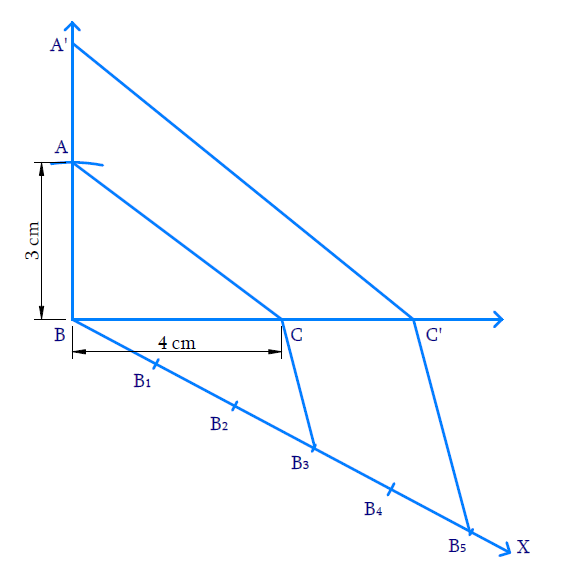Steps of constructions:

(i) Draw $${{BC = 4\, \rm{cm}}}{{.}}$$ At  $$B,$$ make an angle $$\angle {{CBY}} = {90^ \circ }$$ and mark $$A$$ on $$BY$$ such that $${{BA = 3 }}\,{\rm{cm}}{{.}}$$ Join $$A$$ to $$C.$$ Thus $${{\Delta ABC}}$$ is constructed.

(ii) Draw the ray $$BX$$ so that $$\angle {{CBX}}$$ is acute.

(iii) Mark $$5$$ ($$5 > 3$$ in $$\frac{5}{3}$$ ) points $${{{B}}_{{I}}}{{,}}\,{{{B}}_{{2}}}{{,}}\,{{{B}}_{{3}}}{{,}}\,{{{B}}_{{4}}}{{,}}{{{B}}_{{5}}}$$ on $$BX$$ so that

\begin{align}&{{B}}{{{B}}_{{1}}}\\=&{{{B}}_{{1}}}{{{B}}_{{2}}}\\=&{{{B}}_{{2}}}{{{B}}_{{3}}}\\=&{{{B}}_{{3}}}{{{B}}_{{4}}}\\=&{{{B}}_{{4}}}{{{B}}_{{5}}}\end{align}

(iv) Join$${B}_{3}$$ ($$3^\rm{rd}$$ point on $$BX$$ as $$3<5$$) to $$C$$ and draw $${{{B}}_{{5}}}{{C'}}$$ parallel to $${{{B}}_{{3}}}{{C}}$$ so that $${{C'}}$$ lies on the extension of $$BC.$$

(v) Draw $${{C'A'}}$$ parallel to $$CA$$ to intersect of the extension of $$BA$$ at $$A’.$$

Now $${{\Delta BA'C'}}$$ is the required triangle similar to $${{\Delta BAC}}$$ where

\begin{align}\frac{{{{BA'}}}}{{{{BA}}}}{{ = }}\frac{{{{BC'}}}}{{{{BC}}}}{{ = }}\frac{{{{C'A'}}}}{{{{CA}}}}{{ = }}\frac{{{5}}}{{{3}}}\end{align}

Proof:

In $${{\Delta B}}{{{B}}_{{3}}}{{C',}}\,\,{{{B}}_{{3}}}{{C}}\,\,{{||}}\,\,{{{B}}_{{3}}}{{{C}}^{{1}}}$$

Hence by Basic proportionality theorem,

\begin{align} \frac{{{{B}}_{{3}}}{{{B}}_{{5}}}}{{B}{{{B}}_{{3}}}}&{=}\frac{{CC }\!\!'\!\!{ }}{{BC}}{=}\frac{{2}}{{3}} \\ \frac{{CC }\!\!'\!\!{ }}{{BC}} {+1}&=\frac{{2}}{{3}}{+1}\qquad \text{(Adding}\,\,{1)} \\ \frac{{CC }\!\!'\!\!{ +BC}}{{BC}} &{=}\frac{{5}}{{3}} \\ \frac{{BC }\!\!'\!\!{ }}{{BC}}&{=}\frac{{5}}{{3}} \end{align}

Consider $${{\Delta BAC}}$$ and $${{\Delta BA'C'}}$$

$$\angle {{ABC}} = \angle {{A'BC'}} = 90^\circ$$

$$\angle {{BCA}} = \angle {{BC'A'}}$$ (Corresponding angles as $${{CA}}\,\,\,{{||}}\,\,{{C'A'}}$$ )

$$\angle {{BAC}} = \angle {{BA'C'}}$$

By AAA axiom, $${{\Delta BAC}}\,\sim\,{{\Delta BA'C'}}$$

Therefore corresponding sides are proportional,

Hence,

\begin{align}\frac{{{{BA'}}}}{{{{BA}}}}{{ = }}\frac{{{{BC'}}}}{{{{BC}}}}&{{ = }}\frac{{{{C'A'}}}}{{{{CA}}}}\\&{{ = }}\frac{{{5}}}{{{3}}}\end{align}

Download FREE PDF of Chapter-11 Constructions
Constructions | NCERT Solutions
Learn from the best math teachers and top your exams

• Live one on one classroom and doubt clearing
• Practice worksheets in and after class for conceptual clarity
• Personalized curriculum to keep up with school